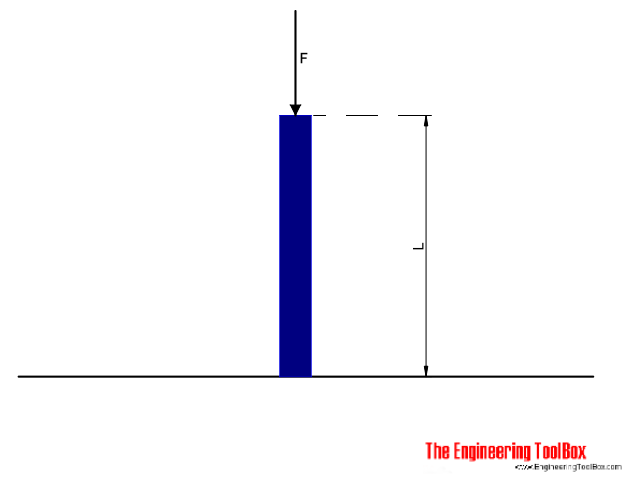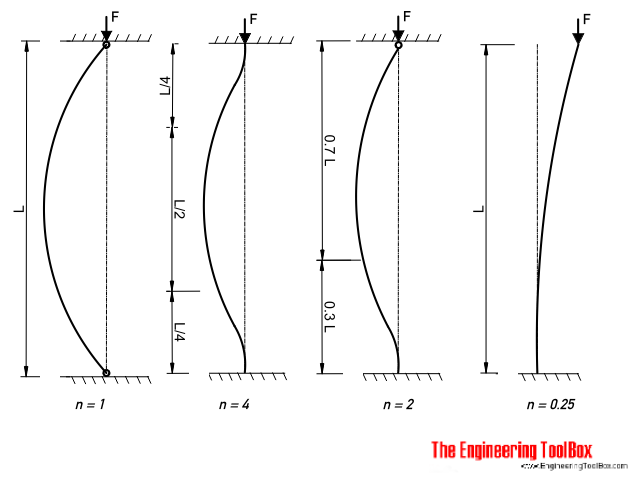Engineering ToolBox - Resources, Tools and Basic Information for Engineering and Design of Technical Applications!

# Euler's Column Formula

## Calculate buckling of columns.Columns fail by buckling when their critical load is reached. Long columns can be analysed with the Euler column formula

F = n π2 E I / L2                             (1)

where

F = allowable load (lb, N)

n = factor accounting for the end conditions

E = modulus of elastisity (lb/in2, Pa (N/m2))

L = length of column (in, m)

I = Moment of inertia (in4, m4)

### Factor Counting for End Conditions• column pivoted in both ends : n = 1
• both ends fixed : n = 4
• one end fixed, the other end rounded : n = 2
• one end fixed, one end free : n = 0.25

### Note!

Equation (1) is sometimes expressed with a k factor accounting for the end conditions:

F = π2 E I / (k L)2                             (1b)

where

k = (1 / n)1/2    factor accounting for the end conditions

 n 1 4 2 0.25 k 1 0.5 0.7 2

### Example - A Column Fixed in both Ends

An column with length 5 m is fixed in both ends. The column is made of an Aluminium I-beam 7 x 4 1/2 x 5.80 with a Moment of Inertia iy = 5.78 in4. The Modulus of Elasticity of aluminum is 69 GPa (69 109 Pa) and the factor for a column fixed in both ends is 4.

The Moment of Inertia can be converted to metric units like

Iy = 5.78 in4 (0.0254 m/in)4

= 241 10-8  m4

The Euler buckling load can then be calculated as

F = (4) π2 (69 109 Pa) (241 10-8  m4) / (5 m)2

=  262594 N

= 263 kN

### Slenderness Ratio

The term "L/r" is known as the slenderness ratio. L is the length of the column and r is the radiation of gyration for the column.

• higher slenderness ratio - lower critical stress to cause buckling
• lower slenderness ratio - higher critical stress to cause buckling
• slenderness ratios L/r < 40: "short columns" where failure mode is crushing (yielding)
• slenderness ratios 40 < L/r < 120: "intermediate columns" where failure mode is a combination of crushing (yielding) and buckling
• slenderness ratio of 120 < L/r < 200: "long columns" where failure mode is buckling

## Related Topics

• ### Beams and Columns

Deflection and stress, moment of inertia, section modulus and technical information of beams and columns.

## Related Documents

• ### Area Moment of Inertia - Typical Cross Sections I

Typical cross sections and their Area Moment of Inertia.
• ### Area Moment of Inertia - Typical Cross Sections II

Area Moment of Inertia, Moment of Inertia for an Area or Second Moment of Area for typical cross section profiles.
• ### British Universal Columns and Beams

Properties of British Universal Steel Columns and Beams.
• ### Concrete Columns

Estimate concrete volume required for concrete columns.
• ### HE-A Steel Beams

Properties of HE-A profiled steel beams.
• ### Mass Moment of Inertia

The Mass Moment of Inertia vs. mass of object, it's shape and relative point of rotation - the Radius of Gyration.
• ### Mild Steel - Square Bars Weight

Typical weights of mild steel square bars.
• ### Radius of Gyration in Structural Engineering

Radius of gyration describes the distribution of cross sectional area in columns around their centroidal axis.
• ### Steel Pipe Columns - Allowable Loads

Allowable concentric loads for steel pipe columns.
• ### Stiffness

Stiffness is resistance to deflection.
• ### Three-Hinged Arches - Continuous and Point Loads

Support reactions and bending moments.
• ### W-Beams - American Wide Flange Beams

Dimensions of American Wide Flange Beams ASTM A6 (or W-Beams) - Imperial units.
• ### Weight of Beams - Stress and Strain

Stress and deformation of vertical beams due to own weight.

• ### Young's Modulus, Tensile Strength and Yield Strength Values for some Materials

Young's Modulus (or Tensile Modulus alt. Modulus of Elasticity) and Ultimate Tensile Strength and Yield Strength for materials like steel, glass, wood and many more.

## Engineering ToolBox - SketchUp Extension - Online 3D modeling!

Add standard and customized parametric components - like flange beams, lumbers, piping, stairs and more - to your Sketchup model with the Engineering ToolBox - SketchUp Extension - enabled for use with older versions of the amazing SketchUp Make and the newer "up to date" SketchUp Pro . Add the Engineering ToolBox extension to your SketchUp Make/Pro from the Extension Warehouse !

We don't collect information from our users. More about

## Citation

• The Engineering ToolBox (2012). Euler's Column Formula. [online] Available at: https://www.engineeringtoolbox.com/euler-column-formula-d_1813.html [Accessed Day Month Year].

Modify the access date according your visit.

9.19.12